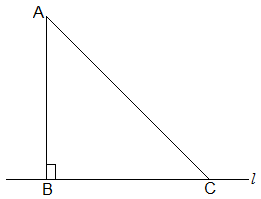Newbie

# Show that of all line segments drawn from a given point not on it, the perpendicular line segment is the shortest. Q.6

• 0

How i solve the important question of ncert class 9th chapter triangles , What is the best solution of question 6 of exercise 7.4 . Please help me to solve this important question in a easiest and simple way.Show that of all line segments drawn from a given point not on it, the perpendicular line segment is the shortest.

Share

1. First, let “l” be a line segment and “B” be a point lying on it. A line AB perpendicular to l is now drawn. Also, let C be any other point on l. The diagram will be as follows:To prove:

AB < AC

Proof:

In ΔABC, B = 90°

Now, we know that

A+B+C = 180°

∴ A +C = 90°

Hence, C must be an acute angle which implies C < B

So, AB < AC (As the side opposite to the larger angle is always larger)

• 0